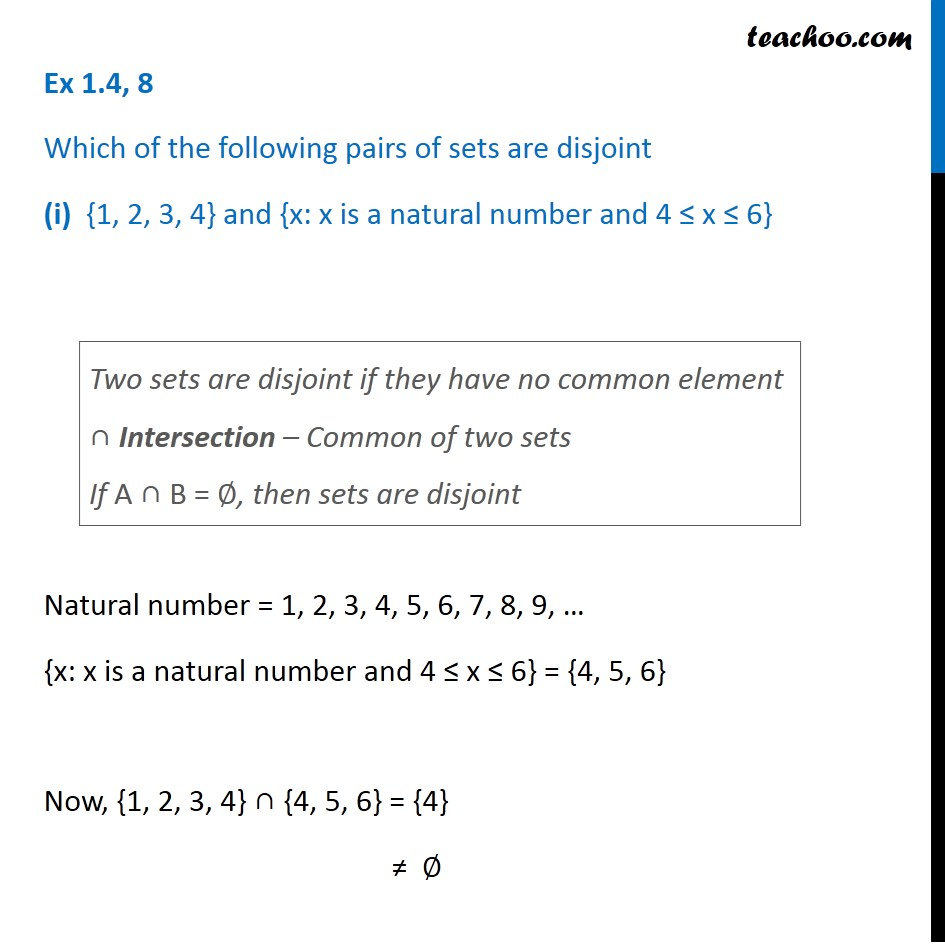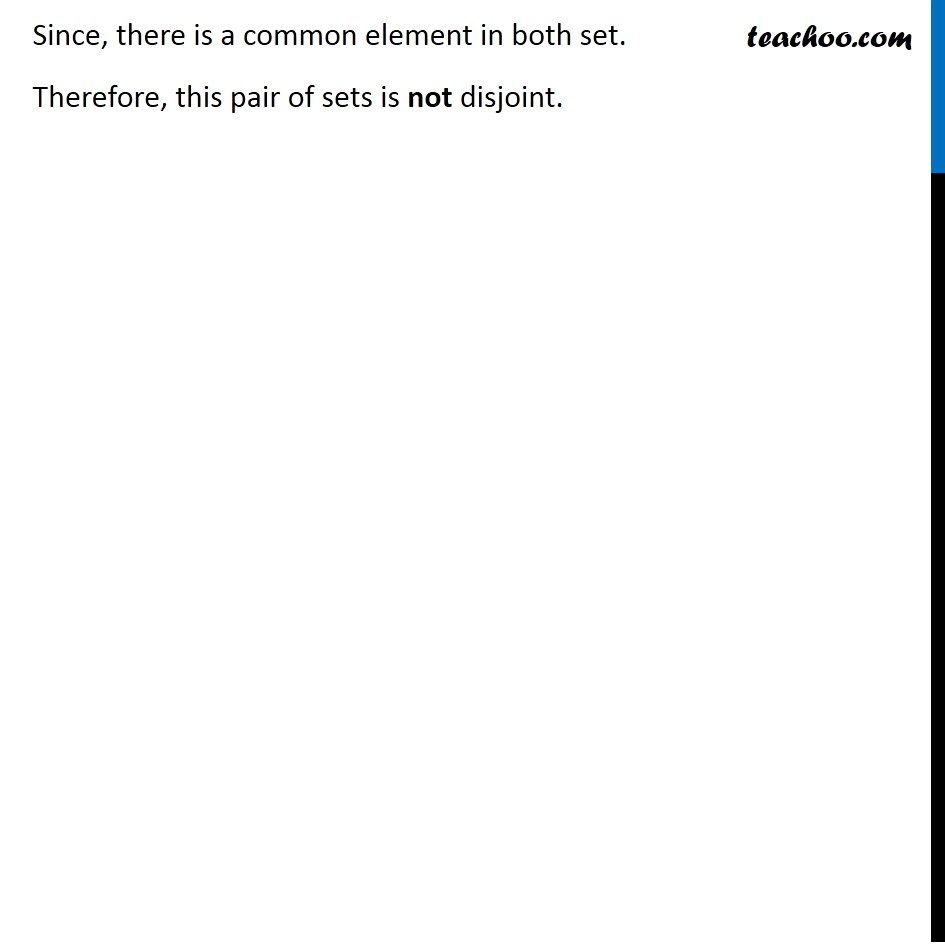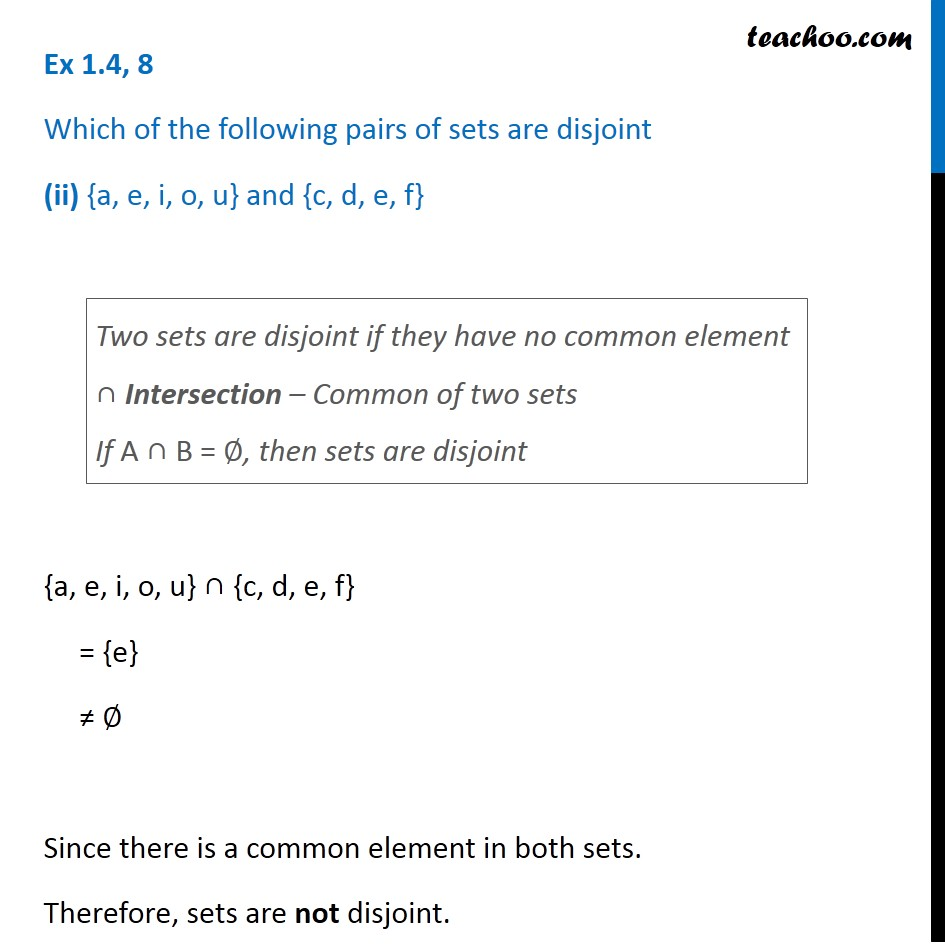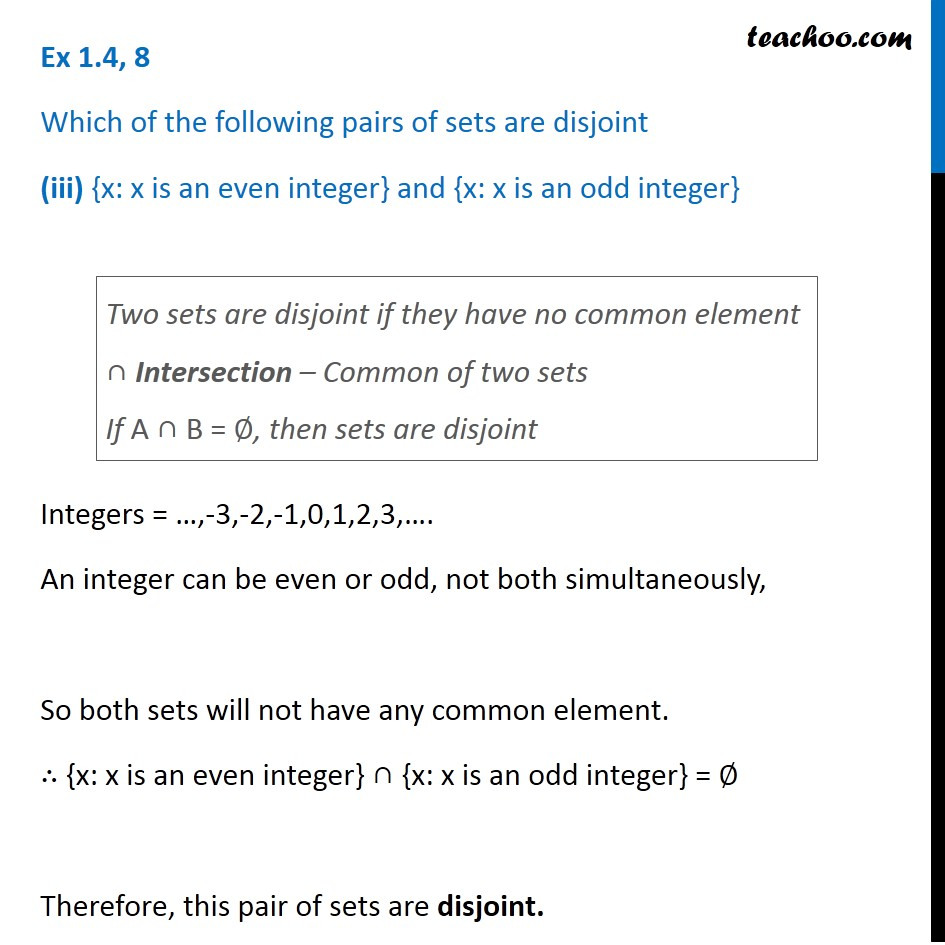1. Chapter 1 Class 11 Sets
2. Serial order wise
3. Ex 1.4

Transcript

Ex 1.4, 8 Which of the following pairs of sets are disjoint (i) {1, 2, 3, 4} and {x: x is a natural number and 4 ≤ x ≤ 6} Natural number = 1, 2, 3, 4, 5, 6, 7, 8, 9, … {x: x is a natural number and 4 ≤ x ≤ 6} = {4, 5, 6} Now, {1, 2, 3, 4} ∩ {4, 5, 6} = {4} ≠ ∅ Two sets are disjoint if they have no common element ∩ Intersection – Common of two sets If A ∩ B = ∅, then sets are disjoint Since, there is a common element in both set. Therefore, this pair of sets is not disjoint. Ex 1.4, 8 Which of the following pairs of sets are disjoint (ii) {a, e, i, o, u} and {c, d, e, f} {a, e, i, o, u} ∩ {c, d, e, f} = {e} ≠ ∅ Since there is a common element in both sets. Therefore, sets are not disjoint. Two sets are disjoint if they have no common element ∩ Intersection – Common of two sets If A ∩ B = ∅, then sets are disjoint Ex 1.4, 8 Which of the following pairs of sets are disjoint (iii) {x: x is an even integer} and {x: x is an odd integer} Integers = …,-3,-2,-1,0,1,2,3,…. An integer can be even or odd, not both simultaneously, So both sets will not have any common element. ∴ {x: x is an even integer} ∩ {x: x is an odd integer} = ∅ Therefore, this pair of sets are disjoint. Two sets are disjoint if they have no common element ∩ Intersection – Common of two sets If A ∩ B = ∅, then sets are disjoint

Ex 1.4

Chapter 1 Class 11 Sets
Serial order wise